# When doing a titration of a 2.310 g sample of bleach, 11.12 mL of a 0.1117...

 When doing a titration of a 2.310 g sample of bleach, 11.12 mL of a 0.1117 M Na2S2O3 solution was used. How many moles of I2 reacted with the Na2S2O3? How many grams of NaOCl must have been present in the solution? (Hint: calculate moles of NaOCl that had to have been present based on the moles of I2 that were found and convert that to grams.) What is the percent by mass of the NaOCl in the bleach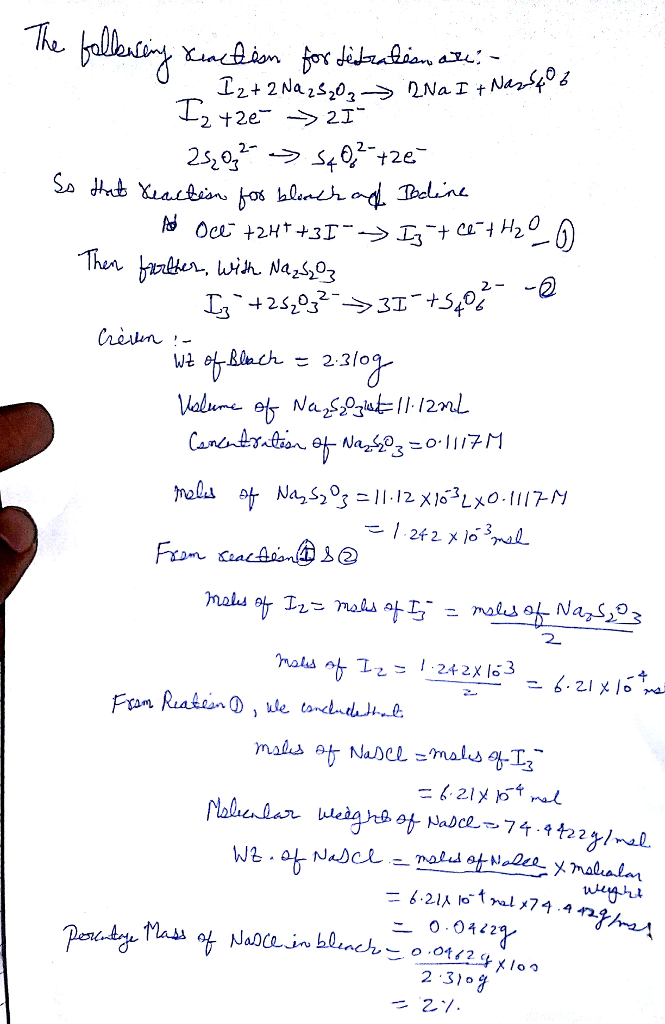##### Add Answer of: When doing a titration of a 2.310 g sample of bleach, 11.12 mL of a 0.1117...
Similar Homework Help Questions
• ### The concentration for Na2S2O4 2. Determining the oxidizing capacity of a household cleanser or liquid bleach...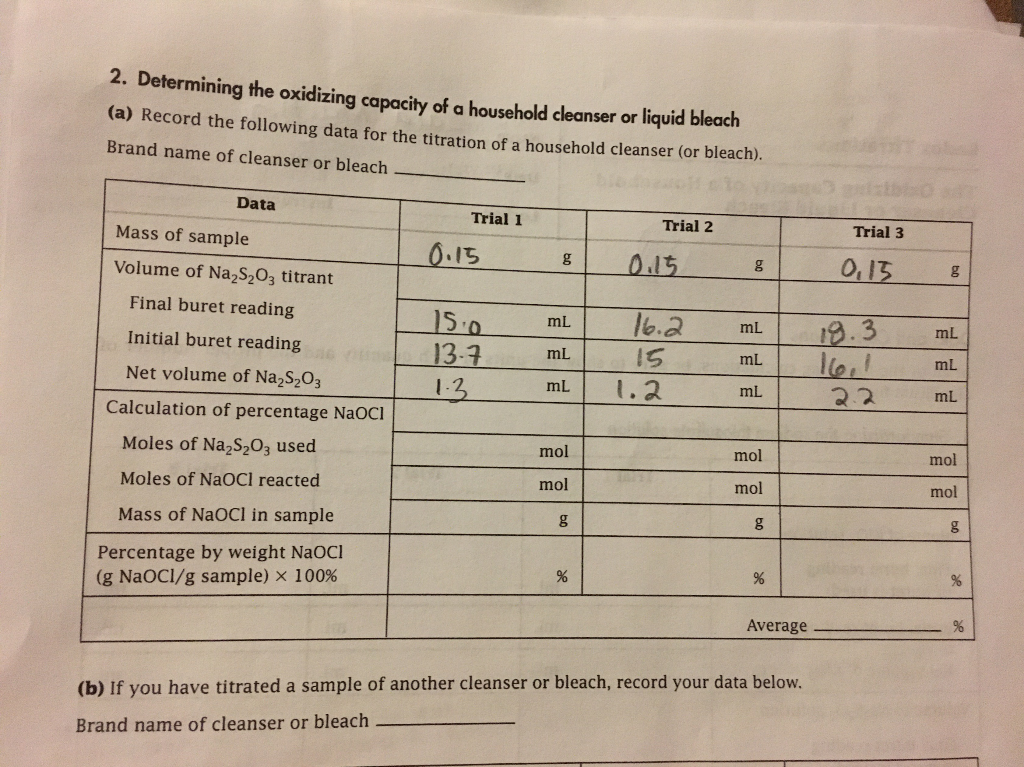The concentration for Na2S2O4 2. Determining the oxidizing capacity of a household cleanser or liquid bleach (a) Record the following data for the titration of a household cleanser (or bleach). Brand name of cleanser or bleach Data Trial 1 Trial 2 Mass of sample Trial 3 O.15 g 015 Volume of NA2S2O3 titrant O,13 g Final buret reading 150 13-7 13 16.2 19.3 lल mL mL mL Initial buret reading mL 15 mL mL Net volume of Na2S2O3 mL ....

• ### Commercial bleach titration

A student diluted 50.00 ml of a commercial bleach to 250.00 ml in a volumetric flask. She took a 20.00 ml aliquot of the solution and reacted it with sodium iodide.Then she added a few drops of starch and titrated the solution with .1000 M Na2S2O3. The blue-black color of the solution disappeared after adding 35.50 ml ofthiosulfate solution. Calculate the number of moles of S2O3 2- used in the titration. Jesus Christ.

• ### when i divide #3 and #4 and then multiply by 100 i get 5.43093385214 and i have to use the correct sig figs. what a...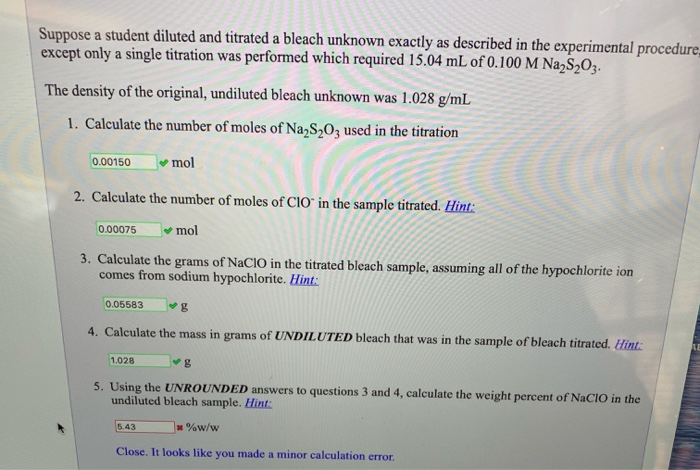when i divide #3 and #4 and then multiply by 100 i get 5.43093385214 and i have to use the correct sig figs. what am i doing wrong? Suppose a student diluted and titrated a bleach unknown exactly as described in the experimental procedure except only a single titration was performed which required 15.04 mL of 0.100 M Na2S2O3. The density of the original, undiluted bleach unknown was 1.028 g/mL 1. Calculate the number of moles of Na2S2O3 used in...

• ### ive tried getting help for these problems numerous times and havent had any luck. Suppose a...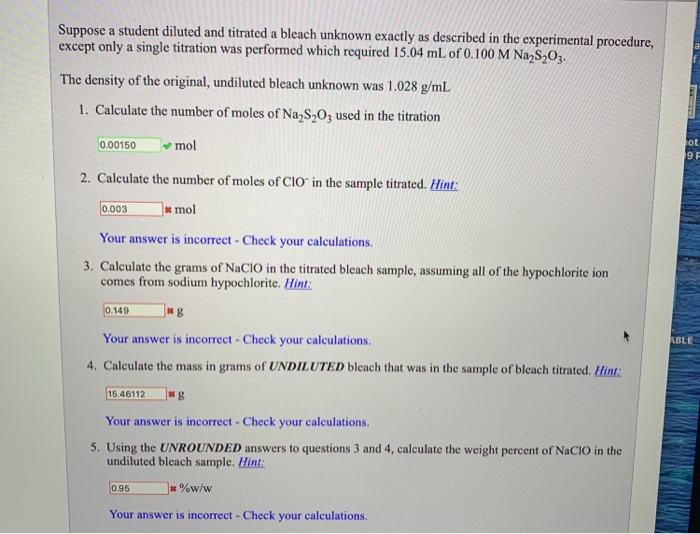ive tried getting help for these problems numerous times and havent had any luck. Suppose a student diluted and titrated a bleach unknown exactly as described in the experimental procedure, except only a single titration was performed which required 15.04 mL of 0.100 M Na S 02. The density of the original, undiluted bleach unknown was 1.028 g/mL 1. Calculate the number of moles of Na2S2O3 used in the titration 0.00150 mol 2. Calculate the number of moles of CIO...

• ### Please answer a-j since this is one question. 3. A student followed the procedure of this...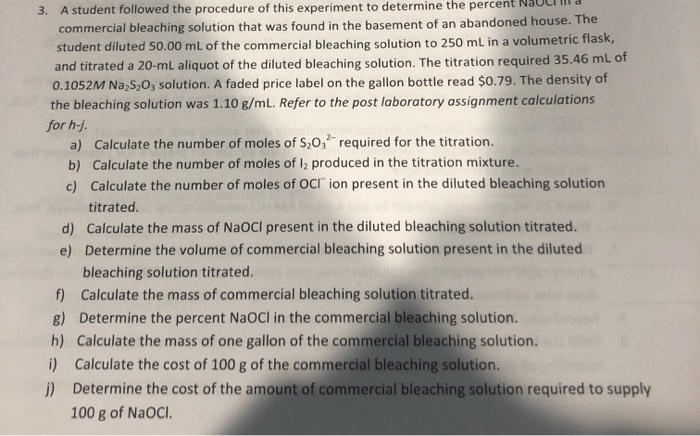Please answer a-j since this is one question. 3. A student followed the procedure of this experiment to determine the percent commercial bleaching solution that was found in the basement of an abandoned house. The student diluted 50.00 mL of the commercial bleaching solution to 250 mL in a volumetric flask, and titrated a 20-mL aliquot of the diluted bleaching solution. The titration required 35.46 mL of 0.1052M Na2S2O3 solution. A faded price label on the gallon bottle read \$0.79....

• ### A 3.00-mL bleach (NaOCl is the active ingredient) sample is mixed with potassium iodide and acid to completely form triiodide (I3 - ) ions. You then titrate the I3 − ions with a thiosulfate (S2O3 2− )...

A 3.00-mL bleach (NaOCl is the active ingredient) sample is mixed with potassium iodide and acid to completely form triiodide (I3 - ) ions. You then titrate the I3 − ions with a thiosulfate (S2O3 2− ) solution you have previously determined to be 0.1306 M. The endpoint is reached when 31.52 mL of the thiosulfate solution is added. What is the molar concentration of the bleach solution? What is its mass percent?

• ### QUESTION 6 The next five problems are calculational in nature. The initial problem statement in each...

QUESTION 6 The next five problems are calculational in nature. The initial problem statement in each case is similar but the numbers will be different. In several cases, you have more information than you need. Be sure to answer only the question asked in each case - don't get carried away. It is found in an attempt to determine the mass percentage of sodium hypochlorite that 14.81 mL of 0.19 M thiosulfate are required to reach the endpoint in the...

• ### 2. A student performs a titration on a 5.00 mL sample of an HBr solution. She...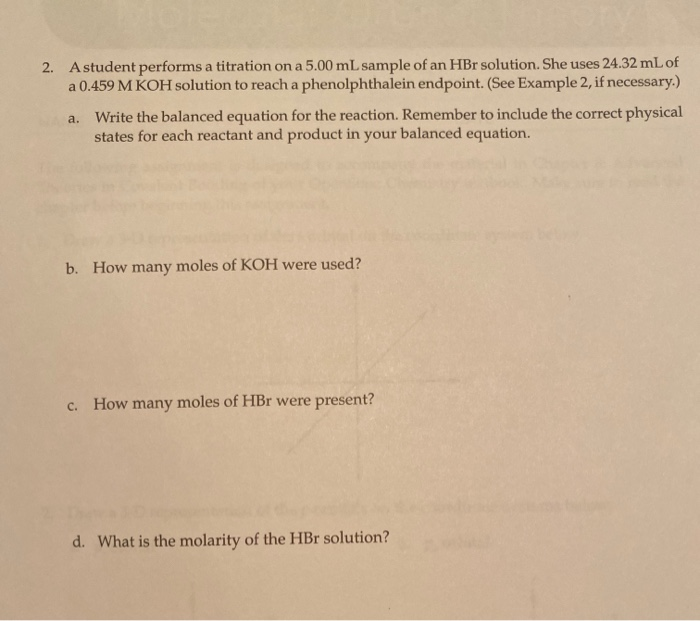2. A student performs a titration on a 5.00 mL sample of an HBr solution. She uses 24.32 mL of a 0.459 M KOH solution to reach a phenolphthalein endpoint. (See Example 2, if necessary.) a. Write the balanced equation for the reaction. Remember to include the correct physical states for each reactant and product in your balanced equation. b. How many moles of KOH were used? C. How many moles of HBr were present? d. What is the molarity...

• ### - Based on your titration data and calculations for determination 1: (1) Calculate the volume of...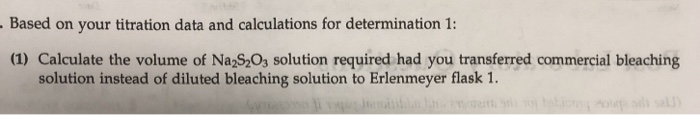- Based on your titration data and calculations for determination 1: (1) Calculate the volume of Na2S2O3 solution required had you transferred commercial bleaching solution instead of diluted bleaching solution to Erlenmeyer flask 1. ANALON16: Determining the Percent Sodium Hypochleise in Commercial Bleaching Solutions Data Sheet brand name of commercial bleaching solution cost of commercial bleaching solution per gallon density of commercial bleaching solution, g mL-' clorca \$2.25 7.05% 10mL olume of commercial bleaching solution diluted to 100 mL, mL...

• ### Acid-Base Titration

Samantha Muir dissolved 0.31 grams of KHP in 25.0 ml of water. She found out that it required 15.5 ml of NaOH to reach the endpoint of titration.a. How many moles of the acid, KHP, were orinignally present before the addition of the base?b. Calculate the concentration of the NaOH solutionc.What is the pH of the solution at the endpoint? (Ka for KHP= 3.89 x 10^-6)

Need Online Homework Help?